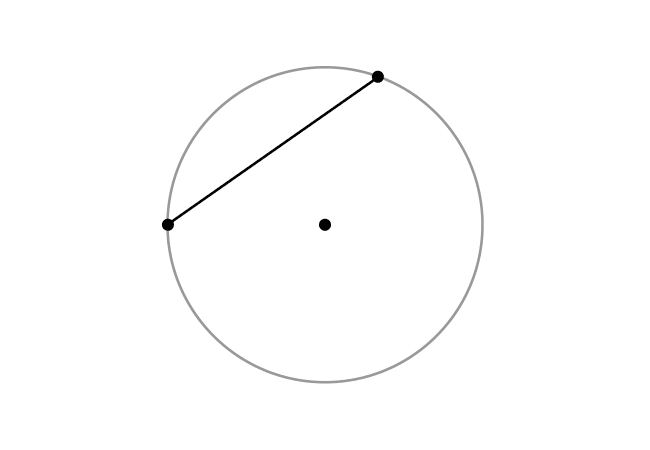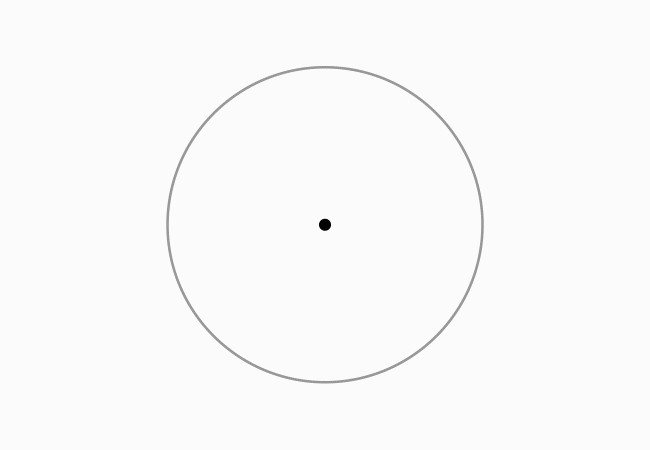# Chord

A line segment whose endpoints lie on a circle is called a chord of a circle.

## IntroductionThere are many points on any circle. In some cases, a point on a circle is connected with another point on the same circle by a straight line.

The two points on the circle become the endpoints of the straight line. Hence, the straight line is geometrically known as a line segment.

The same line segment connects the both points on the circle and it is called a chord of the circle in geometric mathematics.

### Example

The concept of a chord of a circle can be understood from an understandable geometrical example.Let $M$ and $N$ be any two points on a circle, and connect them by a straight line. The points $M$ and $N$ become the endpoints of line segment $\overline{MN}$ geometrically. So, the line segment $\overline{MN}$ is called a chord of this circle.

Similarly, $D$ and $E$ are another two points on the same circle but they are exactly opposite in the point view of the center (or centre) of the circle. The line segment $\overline{DE}$ is also called a chord of the circle.

Actually, the chord $\overline{DE}$ also represents the diameter of circle in this case. It clears that the line segment that represents the diameter is a longest chord of the circle.

Latest Math Topics
Jun 26, 2023
Jun 23, 2023

Latest Math Problems
Jul 01, 2023
Jun 25, 2023
###### Math Questions

The math problems with solutions to learn how to solve a problem.

Learn solutions

Practice now

###### Math Videos

The math videos tutorials with visual graphics to learn every concept.

Watch now

###### Subscribe us

Get the latest math updates from the Math Doubts by subscribing us.